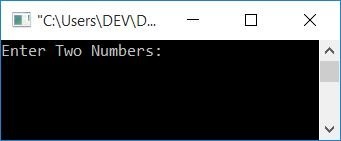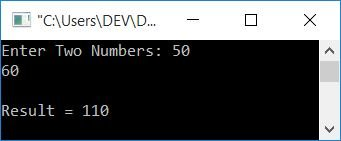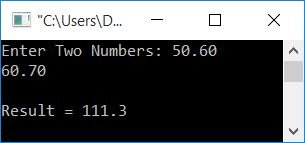# C++ Program to Add Two Numbers

In this article, you will learn and get code about the addition of any two numbers given by the user (at run-time). Here is the list of programs. All of these programs perform the same function, which is to add two numbers entered by the user, but they do so in different ways:

## Add two numbers of the integer type

Let's first start with a very simple program for the addition of two numbers. The dry run of this program is given just after the output.

```#include<iostream>
using namespace std;

int main()
{
cout<<"Enter Two Numbers: ";
cin>>num1>>num2;
cout<<endl;
return 0;
}```

This program was built and runs under the Code::Blocks IDE. Here is the sample run:Now supply any two numbers as input, say 50 and 60. Press ENTER to see the output of the addition of the given two numbers, as shown in the screenshot below:Now let's take a look at the brief explanation of the code along with a dry run using 10 and 20 as input.

• Three variables, namely num1, num2, and add of type int, get declared.
• These variables hold only integer-type values. That is, a whole number:a number that does not contain any decimal points.
• Now ask the user to enter any two numbers.
• Get and store these two numbers in variables num1 and num2.
• Now just initialize num1+num2 to the variable add.
• Finally, print the value of add as output.
• For example, if the user enters 10 and 20 as input, then, 10 gets stored in num1 and 20 gets stored in num2.
• Using num1+num2, 10+20, or 30 gets initialized to add.
• And 30 is the addition result of 10 and 20. That's it.

### What if the user enters real numbers?

The above program is correct only if the user enters the two numbers as integer values. That is, a value that does not contain any decimal parts. But what if the user supplies any value that contains a decimal part? In other words, what if the user enters real numbers? The solution to this problem is given in the following program.

```#include<iostream>
using namespace std;

int main()
{
cout<<"Enter Two Numbers: ";
cin>>num1>>num2;
cout<<endl;
return 0;
}```

As you can see from the above program, To add any two real numbers, just change the data type to float (floating-point type) before declaring all the three variables, say num1, num2, and add. so that these variables become able to handle floating-point values (values that contain decimal parts). Here is a sample run of the above program:## Add two numbers in C++ using a function

Let's use this approach to create a program that adds two numbers given by the user. The output of this program is totally the same as the output of the very first program given over here.

```#include<iostream>
using namespace std;

int main()
{
cout<<"Enter Two Numbers: ";
cin>>num1>>num2;
cout<<endl;
return 0;
}
{
return (a+b);
}```

## Add two numbers in C++ using recursion

This program uses a recursive function to apply the addition of two numbers. A recursive function is one that calls itself from its definition part.

```#include<iostream>
using namespace std;

int main()
{
cout<<"Enter Two Numbers: ";
cin>>num1>>num2;
cout<<endl;
return 0;
}
{
if(b==0)
return a;
else
}```

## Add two numbers in C++ using the friend function

The question is: write a program in C++ that uses the friend function to add two numbers entered by the user. The answer to this question is given below.

```#include<iostream>
using namespace std;

class CodesCracker
{
private:
int a, b, sum;
public:
void getData()
{
cout<<"Enter Two Numbers: ";
cin>>a>>b;
}
void showResult()
{
cout<<"\nResult = "<<sum;
}
};
int main()
{
CodesCracker c;
c.getData();
c.showResult();
cout<<endl;
return 0;
}
{
c.sum = (c.a) + (c.b);
}```

## In C++, use Class to add two numbers

This is the last program of this article; here we have applied the class approach to create a program in C++ for the addition of two given numbers.

```#include<iostream>
using namespace std;

class CodesCracker
{
private:
int x, y;
public:
void getData();
};
void CodesCracker::getData()
{
cout<<"Enter Two Numbers: ";
cin>>x>>y;
}
{
return (x+y);
}
int main()
{
CodesCracker c;
int sum;
c.getData();
cout<<"\nResult = "<<sum;
cout<<endl;
return 0;
}```

#### Same Program in Other Language

You might enjoy the following program in another programming language:

C++ Quiz

« Previous Program Next Program »